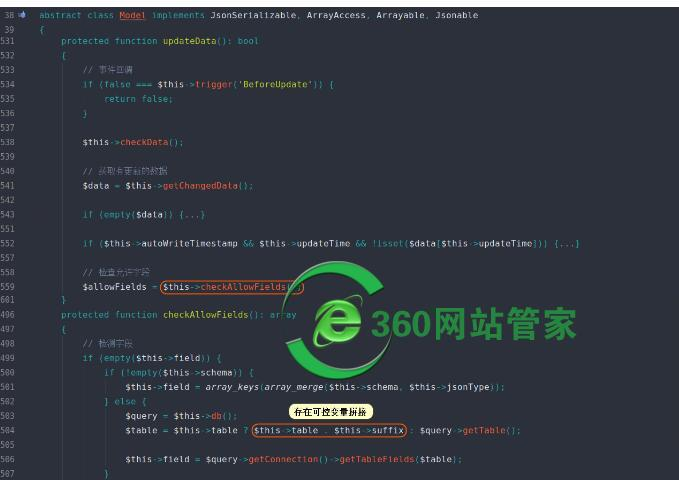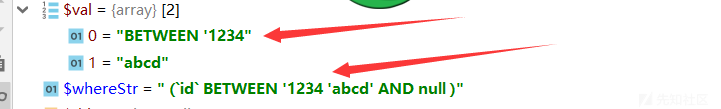• A+

前言CMS 中难免会调用到 SQL 执行（废话），百密总有一疏，今天跟师傅们分享一些平时审计时遇到的注入，可能不全面，希望和师傅们一起学习。

关于 ThinkPHP 中的 注入

ThinkPHP3.2.3

\$User = D('User');
\$user = \$User->where(\$map)->find();
}

public function where(\$where,\$parse=null){

....

if(isset(\$this->options['where'])){
\$this->options['where'] =   array_merge(\$this->options['where'],\$where);
}else{
\$this->options['where'] =   \$where;
}

return \$this;
}

public function select(\$options=array()) {
\$this->model  =   \$options['model'];
\$this->parseBind(!empty(\$options['bind'])?\$options['bind']:array());
\$sql    = \$this->buildSelectSql(\$options); // 生成 sql 语句
\$result   = \$this->query(\$sql,!empty(\$options['fetch_sql']) ? true : false);
return \$result;
}

ThinkPHP5.0

TP5parseWhereItemDb.class.php，但其实也有一段和 3.2 中差不多的代码：

if(isset(\$val) && 'exp'==\$val) {
\$whereStr .= \$key.' '.strtoupper(\$val).' '.\$val;
}• 微信 wzgj360
• 联系免费答疑
•• QQ 613049615
• 联系免费答疑
•